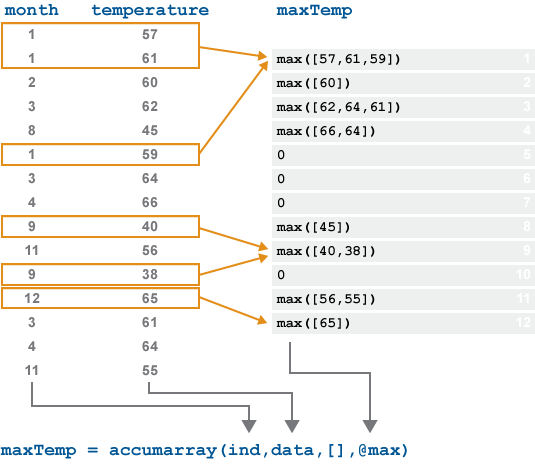# accumarray

## 语法

``B = accumarray(ind,data)``
``B = accumarray(ind,data,sz)``
``B = accumarray(ind,data,sz,fun)``
``B = accumarray(ind,data,sz,fun,fillval)``
``B = accumarray(ind,data,sz,fun,fillval,issparse)``

## 说明

``B = accumarray(ind,data)` 根据 `ind` 中指定的组，通过对向量 `data` 的元素进行累加来对组数据求和。然后计算每组的总和。`ind` 中的值定义数据所属的组以及存储每个组总和的输出数组 `B` 的索引。要按顺序返回组总和，请将 `ind` 指定为向量。然后，对于索引为 `i` 的组，`accumarray` 返回其在 `B(i)` 中的总和。例如，如果 `ind = [1 1 2 2]'` 和 `data = [1 2 3 4]'`，则 `B = accumarray(ind,data)` 返回列向量 `B = [3 7]'`。要以另一种形状返回组总和，请将 `ind` 指定为矩阵。对于 m×n 矩阵 `ind`，其中每一行的值表示指定的组和输出 `B` 的 n 维索引。例如，如果 `ind` 包含 `[3 4]` 形式的两行，则 `data` 中对应元素的总和存储在 `B` 的 (3,4) 元素中。`B` 中未在 `ind` 中显示其索引的元素默认用 `0` 填充。`

``B = accumarray(ind,data,sz)` 返回填充到 `sz` 大小的数组 `B`。将 `sz` 指定为正整数向量，该向量等于或大于 `ind` 中的维度长度。输出中的额外元素用 `0` 填充。将 `sz` 指定为 `[]`，让 `ind` 中的索引确定输出的大小。`

``B = accumarray(ind,data,sz,fun)` 将函数 `fun` 应用于由 `ind` 指定的 `data` 中的每个组。使用 `@` 符号指定 `fun`，如 `@mean`，或指定为 `[]` 以使用默认值 `@sum`。`

``B = accumarray(ind,data,sz,fun,fillval)` 使用标量值 `fillval` 填充未被 `ind` 中的任何索引引用的 `B` 的所有元素。将 `fillval` 指定为 `[]` 以使用默认值 `0`。`

``B = accumarray(ind,data,sz,fun,fillval,issparse)` 返回数组 `B`，当 `issparse` 是 `true` 或 `1` 时，该数组是稀疏数组；如果 `issparse` 是 `false` 或 `0`，则该数组为满数组。默认情况下，输出 `B` 为完全数组。`

## 示例

`data = (1:6)'`
```data = 6×1 1 2 3 4 5 6 ```
`ind = [1 3 4 2 4 1]'`
```ind = 6×1 1 3 4 2 4 1 ```

`ind` 中指定的组对 `data` 中的值求和。

`B = accumarray(ind,data)`
```B = 4×1 7 4 2 8 ```

`B = groupsummary(data,ind,'sum')`
```B = 4×1 7 4 2 8 ```

`ind = [1 1 4 2 4 3]';`

`B = accumarray(ind,1)`
```B = 4×1 2 1 1 2 ```

`B = groupcounts(ind)`
```B = 4×1 2 1 1 2 ```

`data = 1:6`
```data = 1×6 1 2 3 4 5 6 ```
`ind = [1 1; 2 2; 3 2; 1 1; 2 2; 4 1]`
```ind = 6×2 1 1 2 2 3 2 1 1 2 2 4 1 ```

`B1 = accumarray(ind,data)`
```B1 = 4×2 5 0 0 7 0 3 6 0 ```

`B2 = accumarray(ind,data,[4 4])`
```B2 = 4×4 5 0 0 0 0 7 0 0 0 3 0 0 6 0 0 0 ```

`data = [100.1 101.2 103.4 102.8 100.9 101.5]'`
```data = 6×1 100.1000 101.2000 103.4000 102.8000 100.9000 101.5000 ```
`ind = [1 1; 1 1; 2 2; 3 2; 2 2; 3 2]`
```ind = 6×2 1 1 1 1 2 2 3 2 2 2 3 2 ```

`B1 = accumarray(ind,data,[],@var)`
```B1 = 3×2 0.6050 0 0 3.1250 0 0.8450 ```

`A2 = accumarray(ind,data,[],@(x) var(x,1))`
```A2 = 3×2 0.3025 0 0 1.5625 0 0.4225 ```

`data = int8(10:15)`
```data = 1x6 int8 row vector 10 11 12 13 14 15 ```
`ind = [1 1 1; 1 1 1; 1 1 2; 1 1 2; 2 3 1; 2 3 2]`
```ind = 6×3 1 1 1 1 1 1 1 1 2 1 1 2 2 3 1 2 3 2 ```

`B = accumarray(ind,data,[],@(x) sum(x,'native'))`
```B = 2x3x2 int8 array B(:,:,1) = 21 0 0 0 0 14 B(:,:,2) = 25 0 0 0 0 15 ```

`data = 1:10`
```data = 1×10 1 2 3 4 5 6 7 8 9 10 ```
`ind = [1 1; 1 1; 1 1; 1 1; 2 1; 2 1; 2 1; 2 1; 2 1; 2 2]`
```ind = 10×2 1 1 1 1 1 1 1 1 2 1 2 1 2 1 2 1 2 1 2 2 ```

`data` 的元素分组到元胞数组。

`B = accumarray(ind,data,[],@(x) {x})`
```B=2×2 cell array {4x1 double} {0x0 double} {5x1 double} {[ 10]} ```

`B{2,1}`
```ans = 5×1 5 6 7 8 9 ```

`data = (101:106)'`
```data = 6×1 101 102 103 104 105 106 ```
`ind = [1 1; 2 2; 3 3; 1 1; 2 2; 4 4]`
```ind = 6×2 1 1 2 2 3 3 1 1 2 2 4 4 ```

`ind` 中的元素为输出定义一个 4×4 矩阵，但仅引用 16 个元素中的 4 个。默认情况下，输出中的其他 12 个元素为 `0`。用 `NaN` 值而不是 `0` 值填充额外的输出元素。

`B = accumarray(ind,data,[],[],NaN)`
```B = 4×4 205 NaN NaN NaN NaN 207 NaN NaN NaN NaN 103 NaN NaN NaN NaN 106 ```

```data = [34 22 19 85 53 77 99 6]; ind = [1 1; 400 400; 80 80; 1 1; 400 400; 400 400; 80 80; 1 1]```
```ind = 8×2 1 1 400 400 80 80 1 1 400 400 400 400 80 80 1 1 ```

`ind` 中的元素为输出定义一个 400×400 矩阵，但仅引用 160,000 个元素中的 3 个。当 `accumarray` 的输出导致生成一个含低密度非零元素的大型数组时，您可以通过将 `issparse` 选项指定为 `true` 来节省内存，即创建稀疏矩阵而不是满矩阵。

`B = accumarray(ind,data,[],[],[],true)`
```B = (1,1) 125 (80,80) 118 (400,400) 152 ```

## 输入参数

• 如果 `ind` 是向量，则每个元素都指定输出数组的一个索引并定义由 `accumarray` 累加的组。所有元素必须为正整数，并且 `ind` 的长度必须与数据向量的长度匹配。

• 如果 `ind` 是 m×n 矩阵，则每行都指定一个输出的 n 维索引。`ind` 中的第 i 行对应于数据中的第 i 个值，`ind` 的行数必须与数据向量的长度匹配。例如，如果 `ind` 是 3×2 矩阵，则它包含三个二维索引。每行的第一个元素是输出的行索引，第二个元素是列索引。

• 如果 `ind` 是索引向量元胞数组，则每个向量的长度必须相同，并且它们被视为列。

• 如果 `data` 是标量，则其值是扩展标量。

• 如果 `data` 为向量，它的长度必须与索引数组中的行数相同。

`issparse``true``1` 时，填充值必须为 `0``[]`，并且计算函数的输入数据和输出必须均为 `double` 类型。

## 输出参数

• 如果 `ind` 是一个列向量，则 `B` 是长度为 `max(ind,[],1)` 的列向量。

• 如果 `ind` 是具有多列的矩阵，则 `B` 是大小为 `max(ind,[],1)` 的多维数组。

## 详细信息

### 累加元素`month` 中的值都不指向输出的 5、6、7 或 10 位置。默认情况下，这些元素在输出中是 `0`

## 提示

• `accumarray` 的行为类似于函数 `groupsummary``groupcounts`，后者分别用于按组计算汇总统计量和对组中的元素数进行计数。有关 MATLAB® 中的更多分组功能，请参阅数据的预处理

• `accumarray` 的行为还与 `histcounts` 函数的行为类似。

• `histcounts` 使用 bin 边界将连续值分组置入某个一维范围中。`accumarray` 使用 n 维索引对数据进行分组。

• `histcounts` 只能返回 bin 计数和 bin 位置。`accumarray` 可以对数据应用任何函数。

您可以将 `accumarray``data = 1` 结合使用来模拟 `histcounts` 的行为。

• `sparse` 函数也具有与 `accumarray` 类似的累积行为。

• `sparse` 使用二维索引对数据进行分组，而 `accumarray` 使用 n 维索引对数据进行分组。

• 对于索引相同的元素，`sparse` 应用 `sum` 函数（对于 `double` 值）或 `any` 函数（对于 `logical` 值），并在输出矩阵中返回标量结果。`accumarray` 默认求和，但可以将任何函数应用于数据。